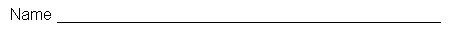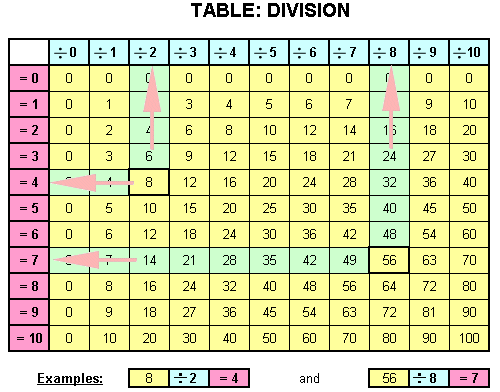Math: How to use the division tableInstructions: 1. Pick a "yellow" number in the table. 2. From the picked "yellow" number, follow the arrow up to the "blue" number. 3. From the picked "yellow" number, follow the arrow to the "pink " number to get the answer. Example: 1. Pick a "yellow" number in the table, example "8". 2. From the picked "yellow" number, follow the arrow to top "blue" row, example "2". 3. From the picked "yellow" number, follow the arrow along the row to the "pink" answer number, example "= 4"